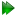Subsections

Rigid body motions for a two domain system

Ball and socket joint

For a molecule consisting of two rigid bodies with pivoted inter-domain or inter-segment motions, the most natural mechanical description of the motion would be that of the spherical joint. This is also known as the ball and socket joint. The mechanical system consists of a single pivot point and three rotational degrees of freedom.

Tilt and torsion angles from robotics

To describe the motional mechanics of a ball and socket joint, the Euler angle system is a poor representation as the angles do not correspond to the mechanical modes of motion. In the field of robotics, many different orientation parameter sets have been developed for describing three degree-of-freedom joint systems. For the spherical joint description of intra-molecular rigid body motions, an angle system for describing symmetrical spherical parallel mechanisms (SPMs), a parallel manipulator, was found to be ideal. This is the tilt-and-torsion' angle system (Bonev and Gosselin, 2006; Huang et al., 1999). These angles were derived independently a number of times to model human joint mechanics, originally as the halfplane-deviation-twist' angles (Korein, 1985), and then as the `tilt/twist' angles (Crawford et al., 1999).

In the tilt-and-torsion angle system, the rigid body is first tilted by the angle θ about the horizontal axis a. The axis a, which lies in the xy-plane, is defined by the azimuthal angle φ (the angle is between the rotated z' axis projection onto the xy-plane and the x-axis). The tilt component is hence defined by both θ and φ. Finally the domain is rotated about the z' axis by the torsion angle σ. The resultant rotation matrix iswhere cη = cos(η) and sη = sin(η) and Rzyz is the Euler rotation in zyz axis rotation notation whereAs σ = α + γ, it can be seen that both these Euler angles influence the torsion angle, demonstrating the problem with this parameterisation.

Modelling torsion

An advantage of this angle system is that the tilt and torsion components can be treated separately in the modelling of domain motions. The simplest model for the torsion angle would be the restriction

 - σmax≤σ≤σmax. (12.76)

The angle can be completely restricted as σmax = 0 to create torsionless models. In this case, the tilt and torsion rotation matrix simplifies toModelling tilt

The tilt angles θ and φ are related to spherical angles, hence the modelling of this component relates to a distribution on the surface of a sphere. At the simplest level, this can be modelled as both isotropic and anisotropic cones of uniform distribution.

Model list

For the modelling of the ordering of the motional frame, the tilt and torsion angle system will be used together with uniform distributions of rigid body position. For the torsion angle σmax, this can be modelled as being rigid ( σmax = 0), being a free rotor ( σmax = π), or as having a torsional restriction ( 0 < σmax < π). For the θ and φ angles of the tilt component, the rigid body motion can be modelled as being rigid ( θ = 0), as moving in an isotropic cone, or moving anisotropically in a pseudo-elliptic cone. Both single and double modes of motion have been modelled. The total list of models so far implemented are:

1. Rigid
2. Rotor
3. Free rotor
4. Isotropic cone
5. Isotropic cone, torsionless
6. Isotropic cone, free rotor
7. Pseudo-ellipse
8. Pseudo-ellipse, torsionless
9. Pseudo-ellipse, free rotor
10. Double rotor

The equations for these models are derived in Chapter 16 on page.

The relax user manual (PDF), created 2020-08-26.